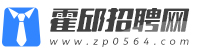• 登录 | 注册• 不限
• 市场销售
• 行政人事
• 城建装修
• 客户服务
• 会计财务
• 普工技工
• 文体培训
• 文职文员
• 设计包装
• 百货零售
• 工厂工业
• 餐饮休闲
• 金融保险
• 管理运营
• 物流贸易
• 司机后勤
• 网络硬件
• 机械仪表
• 咨询顾问
• 电子通讯
• 医疗美容
• 翻译法律
• 轻工工艺
• 化工制药
• 摄影影视
• 能源环保
• 编辑发行
• 其他分类

• 不限
• 财务主管/经理
• 会计
• 会计助理
• 注册会计师
• 注册审计师
• 审计
• 总账主任
• 出纳
• 审计(核)主管
• 统计
• 财务分析
• 成本分析/核算
• 帐目(进出口)管理
• 营运会计经理
• 审计会计经理
• 会计财务其他相关职位

• 不限
• 城关镇
• 河口镇
• 周集镇
• 临水镇
• 新店镇
• 石店镇
• 马店镇
• 孟集镇
• 花园镇
• 扈胡镇
• 长集镇
• 乌龙镇
• 高塘镇
• 龙潭镇
• 岔路镇
• 冯井镇
• 众兴集镇
• 夏店镇
• 曹庙镇
• 范桥镇
• 潘集镇
• 彭塔乡
• 王截流乡
• 临淮岗乡
• 城西湖乡
• 宋店乡
• 三流乡
• 邵岗乡
• 白莲乡
• 冯瓴乡
• 经济开发区
• 六安市区
• 其他地区

• 不限
• 今天
• 三天内
• 一周内
• 一个月内
• 三个月内

• 不限
• 全职
• 兼职

• 不限
• 1000元以下
• 1000-2000元
• 2000-3000元
• 3000-5000元
• 5000-8000元
• 8000-1.2万元
• 1.2万-2万元
• 2万元以上
• 面议